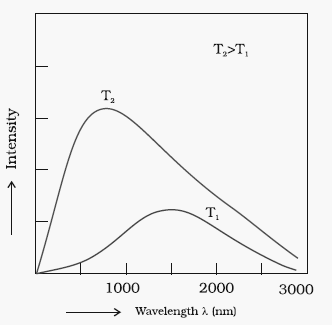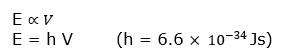# Planck Quantum Theory|Quantization Of Energy

## Plancks quantum theory

### Introduction

Maxwell Theory of Electromagnetic waves or radiation was able to explain diffraction and interference but it was not able to explain the nature of emission of radiation from hot bodies (black -body radiation), ejection of electrons from metal surface when radiation strikes it (photoelectric effect), variation of heat capacity of solids as a function of temperature

Hot objects emit electromagnetic radiations over a wide range of wavelengths.Intensities of radiations of different wavelengths emitted by hot body depend upon its temperature. Also when the surface of an object is irradiated with light (electromagnetic radiation), a part of radiant energy is generally reflected as such, a part is absorbed and a part of it is transmitted.

The ideal body which emit and absorbs all the frequencies is called Black body and the radiation emitted by such body is called Black body radiation.
The exact frequency distribution of emitted radiations from black body depends only on its temperature.

Also a black body is in thermal equilibrium with its surroundings. It radiates same amount of energy per unit area as it absorbs from its surrounding in any given time. The amount of light emitted (intensity of radiation) from a black body and its spectral distribution depends only on its temperature. Also At a given temperature, intensity of radiation emitted increases with the increase of wavelength, reaches a maximum value at a given wavelength and then starts decreasing with further increase of wavelength, as shown in below figure.Also, as the temperature increases,maxima of the curve shifts to short wavelength. Several attempts were made to predict the intensity of radiation as a function of wavelength, but Scientist was not able to explain all these with the theory of electromagnetic waves

### Planck's Theory

Planck was able explain the phenomenon of black body radiation by assumption that absorption and emmission of radiation arises from oscillator i.e., atoms in the wall of black body. Their frequency of oscillation is changed by interaction with oscilators of electromagnetic radiation
This theory has following traits: -
(a) The radiation energy emitted or observed in the form of small packets of energy(in discrete quantities and not in a continuous manner). Such packets are known as quantum or photon.
(b) Energy of each photon is directly proportional to frequency of radiation.Where ‘h’ is planck’s constant.

### Quantization Of Energy

• The restriction of any property to discrete values is called quantization.
• Planck's Quantisation can be understood by comparing it to person standing on a staircase. A person can stand on any step of a staircase, but it is not possible for him/her to stand in between the two steps. The energy can take any one of the values from the following set, but cannot take on any values between them.
$E = 0, h \nu, 2h \nu, 3h \nu ....nh \nu .....$

Question 1
Calculate energy of one mole of photons of radiation whose frequency is $5 \times 10^{14}$ Hz.
Solution
Energy (E) of one photon is given by the expression
$E = h \nu$
$h = 6.626 \times 10^{-34}$ J s
$\nu = 5 \times 10^{14} \; s^{-1}$ (given)
Hence energy of one photon
$E = (6.626 \times 10^{-34}) \times (5 \times 10^{14})$
$= 3.313 \times 10^{-19}$ J
Energy of one mole of photons
$= (3.313 \times 10^{-19}) \times (6.022 \times 10^{23})$
= 199.51 kJ

Question 2
A 200 watt bulb emits monochromatic light of wavelength 400 nm. Calculate the number of photons emitted per second by the bulb.
Solution
Power of the bulb = 200 watt =200 J/s
$\lambda= 400 \; nm= 400 \times 10^{-9}$ m
Energy of one photon is given by
$E = h \nu = \frac {hc}{\lambda}$
$=\frac {6.626 \times 10^{-34} \times 3 \times 10^{8}}{400 \times 10^{-9}}$
$= 4.969 \times 10^{-19}$ J
Number of photons emitted
$=\frac {200}{4.969 \times 10^{-19}}$
$=4.024 \times 10^{20}$ /sec

Question 3
The Planck's constant h has the dimensions equal to
(a) $[ML^2T^{-2}]$
(b)$[MLT^{-2}]$
(c) $[ML^2T^{-1}]$
(d) $[MLT]$
Solution
(c)

Question 4
Wavelengths of different radiations are given below :
$\lambda (A)= 300$ nm
$\lambda(B)= 300$ m
$\lambda(C)= 3$ nm
$\lambda (D)= 30 \; A^0$
Which one has the highest Energy
(i) A
(ii) B
(iii) C
(iv) D
Solution
(iii) and (iv)
as $E \alpha \frac {1}{\lambda}$

Question 5
Which one has the least Energy
(i) A
(ii) B
(iii) C
(iv) D
Solution
(ii)

Question 6
What is the packet of energy called?
(i) Electron
(ii) Photon
(iii) Positron
(iv) Proton
Solution
(ii)